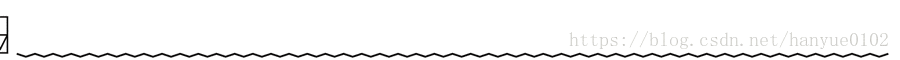# 欢迎大家积极参与ACM训练计划中~## 简述

(等过了一段时间后，题解就只用C++来写了，目前先把C的基础打牢，然后慢慢过渡到C++，C++版本的也可以动手敲一敲代码)

# A

A - X Cubic

C语言版本的就不作解释了，详情看代码。

pow() 函数用来求 x 的 y 次幂（次方），x、y及函数值都是double型 ，其原型为：
double pow(double x, double y);
pow()用来计算以x 为底的 y 次方值，然后将结果返回。设返回值为 ret，则 ret = xy。
(c语言后面也会讲到，详情可百度查阅更多知识)

C语言版本

#include<stdio.h>
int main(){
int x;
scanf("%d",&x);
x=x*x*x;
printf("%d\n",x);
return 0;
}


C++版本

#include<bits/stdc++.h>  //万用头文件
using namespace std;
int main(){
ios::sync_with_stdio(false);
cin.tie(0);
int x;
cin>>x;
cout<<(int)pow(x,3)<<endl;
return 0;
}


ios::sync_with_stdio(false);//常用加速模板
cin.tie(0);


# B

B - Rectangle

C语言版本

#include<stdio.h>
int main(){
int a,b;
scanf("%d %d",&a,&b);
printf("%d %d\n",a*b,(a+b)*2);
return 0;
}


C++版本

#include<bits/stdc++.h>
using namespace std;
int main(){
ios::sync_with_stdio(false);
cin.tie(0);
int a,b;
cin>>a>>b;
cout<<a*b<<" "<<(a+b)*2<<endl;
return 0;
}


# C

C - Watch

C语言版本

#include<stdio.h>
int main()
{
int S,h,m,s;
scanf("%d",&S);
h = S/3600;
m = S%3600/60;
s = S%3600%60;
printf("%d:%d:%d\n",h,m,s);
return 0;
}


C++版本

#include<bits/stdc++.h>
using namespace std;
int main(){
int S,h,m,s;
cin>>S;
h = S/3600;
m = S%3600/60;
s = S%3600%60;
cout<<h<<":"<<m<<":"<<s<<endl;
return 0;
}


# D

D - Small, Large, or Equal

C语言版本

#include<stdio.h>
int main(){
int a,b;
scanf("%d %d",&a,&b);
if(a<b)
printf("a < b\n");
else if(a>b)
printf("a > b\n");
else
printf("a == b\n");
return 0;
}


C++版本

#include<bits/stdc++.h>
using namespace std;
int main(){
int a,b;
cin>>a>>b;
if(a<b)
cout<<"a < b"<<endl;
else if(a>b)
cout<<"a > b"<<endl;
else
cout<<"a == b"<<endl;
return 0;
}


# E

E - Range

&&符号运用

C语言版本

#include<stdio.h>
int main(){
int a,b,c;
scanf("%d %d %d",&a,&b,&c);
if(a<b&&b<c)
printf("Yes\n");
else
printf("No\n");
return 0;
}


C++版本

#include<bits/stdc++.h>
using namespace std;
int main(){
int a,b,c;
cin>>a>>b>>c;
if(a<b&&b<c)
cout<<"Yes"<<endl;
else
cout<<"No"<<endl;
return 0;
}


## 结尾学如逆水行舟，不进则退


JS，TS，LeetCode，Vue，React，算法爱好者。

07-1952
07-0681705-11149
06-15499
05-21199
03-221385
05-21387
08-11479
05-20155
10-08529点击重新获取扫码支付余额充值